# Difference between revisions of "Intra"

In general, intra-frame predictors are coefficients that are used to predict the contents of a block based on its three neighboring blocks. Consider a 4x4 block structure: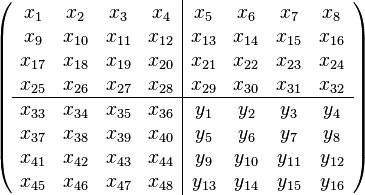$\left( \begin{array}{cccc|cccc} x_{1}&x_{2}&x_{3}&x_{4}&x_{5}&x_{6}&x_{7}&x_{8}\\ x_{9}&x_{10}&x_{11}&x_{12}&x_{13}&x_{14}&x_{15}&x_{16}\\ x_{17}&x_{18}&x_{19}&x_{20}&x_{21}&x_{22}&x_{23}&x_{24}\\ x_{25}&x_{26}&x_{27}&x_{28}&x_{29}&x_{30}&x_{31}&x_{32}\\ \hline x_{33}&x_{34}&x_{35}&x_{36}&y_{1}&y_{2}&y_{3}&y_{4}\\ x_{37}&x_{38}&x_{39}&x_{40}&y_{5}&y_{6}&y_{7}&y_{8}\\ x_{41}&x_{42}&x_{43}&x_{44}&y_{9}&y_{10}&y_{11}&y_{12}\\ x_{45}&x_{46}&x_{47}&x_{48}&y_{13}&y_{14}&y_{15}&y_{16}\\ \end{array} \right)$

Assuming a linear predictor, we would like to find the 768 coefficients that best predict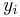$y_i$ from the neighboring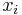$x_i$. That is, for a given block with coefficients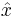$\hat x$ and$\hat y$, we would like a 48x16 matrix$\beta$ such that the residual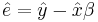$\hat e=\hat y-\hat x \beta$ is minimized (for some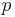$p$-norm). Because it is this residual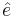$\hat e$ that is quantized and coded in the bitstream, we would prefer that most of its coefficients are zero. This will be discussed further when comparing the different$p$-norms.

## Block Modes

These predictors often follow certain geometry in the time-domain image space. Imagine a vertical edge that runs through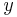$y$. A natural predictor might be to simply copy the values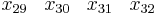$\begin{array}{cccc}x_{29}&x_{30}&x_{31}&x_{32}\end{array}$ down into each row of the 4x4 block$y$. In order to account for the different possible geometries, each block is assigned a mode which indicates what set of prediction coefficients to use (the$\beta$). When encoding a block$y$, the encoder chooses a mode, computes$\hat e$ and writes both the block mode and the residual$\hat e$.

Because the decoder must know the coefficients for each of the block modes, there is a tradeoff between how well we can predict the values in$y$ (number of block modes) with decoder complexity. Our hypothesis is that the optimal number of block modes will correspond to how closely we can fit different geometries inside the block. For 4x4 blocks, this might correspond to a mode for each of the 8 cardinal directions (ask Jason to clarify) with larger blocks potentially supporting a larger number of directions. In addition, we would like to support the DC and True Motion modes from Theora/VP3 and WebM/VP8 as they are often the best fit. For common video sequences, In the case of True Motion, anywhere from 20% to 45% of the intra frames use this mode are

This is slightly complicated by the fact that our coefficients are not in the time-domain, but are the result of a lapped transform, see TDLT. In practice all of these modes have an equivalent in the frequency domain (even True Motion?). As a starting point, we are using the 10 modes from Theora to classify the blocks from a set of sample images. Each category of blocks will be used to construct a set of predictors$\beta$ which is then used to reclassify the blocks based on Sum of Absolute Transform Differences (SADT). Tim suggested weighting each blocks contribution based on SADT_bestfit-SADT_nearest.

##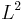$L^2$-norm

Suppose for some mode we have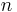$n$ blocks. We can then construct the matrices$X$ and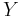$Y$ where the ith row of each contains the zero-mean values from the ith block.$X=\begin{pmatrix} x_{1,1}-\bar{x_1} & x_{1,2}-\bar{x_2} & \cdots & x_{1,m}-\bar{x_m}\\ x_{2,1}-\bar{x_1} & x_{2,2}-\bar{x_2} & \cdots & x_{2,m}-\bar{x_m}\\ \vdots & \vdots & \ddots & \vdots \\ x_{n,1}-\bar{x_1} & x_{n,2}-\bar{x_2} & \cdots & x_{n,m}-\bar{x_m} \end{pmatrix}$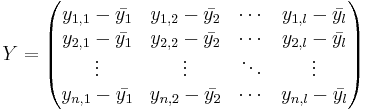$Y=\begin{pmatrix} y_{1,1}-\bar{y_1} & y_{1,2}-\bar{y_2} & \cdots & y_{1,l}-\bar{y_l}\\ y_{2,1}-\bar{y_1} & y_{2,2}-\bar{y_2} & \cdots & y_{2,l}-\bar{y_l}\\ \vdots & \vdots & \ddots & \vdots \\ y_{n,1}-\bar{y_1} & y_{n,2}-\bar{y_2} & \cdots & y_{n,l}-\bar{y_l} \end{pmatrix}$

In the case of 4x4 blocks,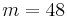$m=48$ and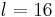$l=16$. Let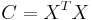$C=X^T X$ and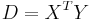$D=X^T Y$. Then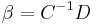$\beta=C^{-1} D$.